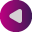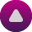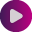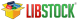Integer ConstantsInteger Constants

Integer constants can be decimal (base 10), hexadecimal (base 16), binary (base 2), or octal (base 8). In the absence of any overriding suffixes, the data type of an integer constant is derived from its value.

Long and Unsigned Suffixes

The suffix L (or l) attached to any constant forces that constant to be represented as a long. Similarly, the suffix U (or u) forces a constant to be unsigned. Both L and U suffixes can be used with the same constant in any order or case: ul, Lu, UL, etc.
Also, there are ULL (or ull) and LL (or ll) suffixes which force constant to be unsigned long long or long long type.

In the absence of any suffix (U, u, L, l, LL, ll, ULL or ull ), a constant is assigned the “smallest” of the following types that can accommodate its value: short, unsigned short, int, unsigned int, long int, unsigned long int, long long or unsigned long long.

Otherwise:

• If a constant has the U suffix, its data type will be the first of the following that can accommodate its value: unsigned short, unsigned int, unsigned long int.
• If a constant has the L suffix, its data type will be the first of the following that can accommodate its value: long int, unsigned long int.
• If a constant has both L and U suffixes, (LU or UL), its data type will be unsigned long int.
• If a constant has the LL, its data type will be long long.
• If a constant has the ULL, its data type will be unsigned long long.

Decimal

Decimal constants from -9223372036854775808 to 18446744073709551615 are allowed. Constants exceeding these bounds will produce an “Out of range” error. Decimal constants must not use an initial zero. An integer constant that has an initial zero is interpreted as an octal constant. Thus,

int i = 10;   /* decimal 10 */
int i = 010;  /* decimal 8  */
int i = 0;    /* decimal 0 = octal 0 */

In the absence of any overriding suffixes, the data type of a decimal constant is derived from its value, as shown below:

Value Assigned to Constant Assumed Type
< -9223372036854775808 Error: Out of range!
-9223372036854775808 – -2147483649 long long
-2147483648 – -32769 long
-32768 – -129 int
-128 – 127 short
128 – 255 unsigned short
256 – 32767 int
32768 – 65535 unsigned int
65536 – 2147483647 long
2147483648 – 4294967295 unsigned long
4294967296 – 18446744073709551615 unsigned long long
> 18446744073709551615 Error: Out of range!

All constants starting with 0x (or 0X) are taken to be hexadecimal. In the absence of any overriding suffixes, the data type of an hexadecimal constant is derived from its value, according to the rules presented above. For example, 0xC367 will be treated as unsigned int.

Binary

All constants starting with 0b (or 0B) are taken to be binary. In the absence of any overriding suffixes, the data type of an binary constant is derived from its value, according to the rules presented above. For example, 0b11101 will be treated as short.

Octal

All constants with an initial zero are taken to be octal. If an octal constant contains the illegal digits 8 or 9, an error is reported. In the absence of any overriding suffixes, the data type of an octal constant is derived from its value, according to the rules presented above. For example, 0777 will be treated as int.

Find them on